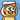# Search Algorithm Summarization in CZ3005 Arificial Intelligence

April 19, 2019

It’s been quite a while since my last update because I am quite busy preparing for my final exams in this semester. Today I would like to share with you the summarization of search algorithms that are exambable in this subject, CZ3005 Artificial Intelligence.

Please note that the algorithms are explained in point form and all the illustrations are extracted from the lecture slides provided by School of Computer Science and Engineering in Nanyang Technological University.

# Definitions

State: State is a “black box” - any arbitrary data structure that supports three problem-specific routines 1. Successor function 2. Goal test 3. Heuristic function Optimal: A search algorithm is optimal if it is the best one (e.g. the shortest), when it finds a solution. Heuristic: A search heuristic h(n) is an estimate of the cost of the shortest path from node n to a goal node. Admissible Heuristic: A search heuristic h(n) is admissible if it is never an overestimate of the cost from n to a goal. b: Maximum forward branching factor m: Maximum path length

# Theorem

• If A* selects a path p as the solution, p is the lowest-cost path.

# Uninformed Search Strategies

Uninformed search strategies do not consider any information about the goal states to decide which path to expand first on the frontier.

• Uniform-cost search
• Depth-first search
• Depth-limited search
• Iterative deepening search

• Treat frontier as a queue [p1, p2, …, pn]
• Illustration
• BFS is complete BFS is guaranteed to find the path that involves the fewest arcs.
• BFS is optimal
• Time Complexity O(b^m)
• Space Complexity O(b^m)
• When is BFS appropriate? _ Space is not a problem _ It’s necessary to find the solution with the fewest arcs * Although all solutions may not be shallow, some are
• When is BFS inappropriate _ Space is limited _ All solutions tend to be located deep in the tree * The branching factor is very large (a node has too many children, so that the deeper level cannot be quickly visited)

## Uniformed-Cost Search

• Treat frontier as a priority queue
• Behaves like BFS
• Sometimes there are costs associated with arcs The cost of the path is the sum of the costs of its arcs.

## Depth-first Search

• Treat frontier as a stack
• Illustration
• DFS is NOT complete If there are cycles in the graph, DFS may get “stuck” in one of them.
• DFS is NOT optimal It can “stumble” on longer solution paths before it gets to shorter ones.
• Time Complexity: O(b^m)
• Space Complexity: O(bm)
• When is DFS appropriate? _ Space is restricted (Complex state representation e.g. robotics) _ There are many solutions, perhaps with long path lengths, particularly for the case in which all paths lead to a solution
• When is DFS inappropriate _ Cycles _ There are shallow solutions.

## Depth-limited Search

• To avoid infinite loops, DFS with a cutoff on the max depth l of a path.
• DLS is complete only if l >= d If l is smaller than d, the deepest nodes cannot be visited)
• DLS is NOT optimal
• Time Complexity: O(b^l)
• Space Complexity: O(bl)

## Iterative Deepening Search

• Re-compute elements of the frontier rather than saving them Iteratively estimate the max depth l of DLS one-by-one If a solution cannot be found at depth D, look for a solution at depths D+1
• Illustration
• IDS is complete
• Time Complexity: O(b^d)
• Space Complexity: O(bd)
• Optimal: Yes
• Preferred method when d is not known.

# Informed Search Strategies

1. A.K.A Heuristic Search
2. There is extra knowledge in the problem that can be used to guide the search: an estimate of the distance from node n to a goal node. This is called a heuristic.
3. There is never a path from n to a goal that has path cost less than h(n).

## Greedy Best-first Search

• Expand the node that appears closest to the goal.
• Use only heuristic function: f(n) = g(n) (estimate of cost from n to g)
• Greedy Search is NOT optimal.
• Greedy Search is NOT complete.
• Time Complexity: O(b^m)
• Space Complexity: O(b^m) because it keeps all nodes in memory

## A* Search

• A* treats the frontier as a priority queue ordered by f(p) = cost(p) + h(p) It always selects the node on the frontier with the lowest estimated total distance.
• If the heuristic is completely uninformative and the edge costs are all the same, A* is equivalent to BFS.
• A* Search is optimal when 1. The branching factor is finite 2. arc costs are strictly positive 3. h(n) is an underestimate of the length of the shortest path from n to a goal node, and is non-negative
• A* Search is complete
• Time Complexity: O(b^m)
• Space Complexity: O(b^m), like BFS, A* maintains a frontier which grows with the size of the tree
• Key advantage of A*: It is optimal and avoids expanding unnecessary paths.

# SummaryWritten by Yi Zhiyue
A Software Engineer · 山不在高，有仙则灵